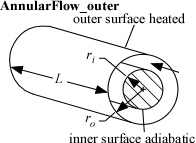Contents - Index

AnnularFlow_outerProcedure AnnularFlow_outer(Fluid\$, T, P, m_dot, r_i, r_o, L, RelRough: h_T, h_H, DELTAP, Nusselt_T,  f, Re) returns the lower and upper bounds on the convection coefficient for developing or fully-developed flow in an annular duct with the outer tube heated.  The inner wall of the annulus is assumed to be insulated so heat transfer occurs across the outer wall.  The area used for heat transfer should be the area of the outer tubel.  The procedure also returns the friction factor for these conditions.

Inputs:

Fluid\$ can be any fluid in the EES database.  The fluid can be an ideal gas, real fluid, brine or fluid in the Solid-Liquid property library.

T - the bulk temperature of the fluid [C], [K], [F], or [R].

P -  pressure can be in [Pa], [kPa], [bar], [MPa], [atm], or [psia].   (Note that for brines, this parameter provides the concentration in % instead of the pressure)

m_dot  -  mass flow rate in [kg/s] or [lbm/hr]

r_i - inner radius of annulus [m] or [ft]

r_o - outer radius of annulus [m] or [ft]

L - length of the annulus [m] or [ft]

RelRough - the ratio of the dispersions on the walls of the duct to the hydraulic diameter  of the duct (must be between 0 and 0.05).  The hydraulic diameter is 2 (r_o - r_i).

Outputs:

h_T -  lower bound heat transfer coefficient in [W/m^2-K] or [Btu/hr-ft^2-R] assuming that the outer wall is at constant temperature

h_H -  upper bound on heat transfer coefficient in [W/m^2-K] or [Btu/hr-ft^2-R] assuming a constant heat flux at the outer wall

DELTAP - pressure difference between the inlet and outlet of the annular space in the pressure units set in the EES Unit System dialog

Nusselt_T - Nusselt number corresponding to the lower heat transfer coefficient.l

f -  friction factor [-]

Re - Reynolds number

Notes:

This procedure calculates the Reynolds and Prandtl numbers and then calls AnnularFlow_outer_ND which determines the Nusselt numbers based on boundary conditions of constant temperature and constant heat flux individually.

Two values of the heat transfer coefficient are returned that should provide upper and lower bounds.  These heat transfer coefficients are both for the outer annular wall with an insulated inner wall.  For turbulent flow, these values are identical.

The procedure will determine if the flow is laminar or turbulent.  Transitional flow is assumed to occur for Reynold's numbers between 2300 and 3000 and interpolation is applied between the laminar and turbulent correlations.

Example 1:

\$unitSystem SI Pa K J

T=363 [K] "average temperature of fluid in duct"

P=101300 [Pa] "pressure of air in annulus"

m_dot=0.001 [kg/s]   "flow rate"

L=5 [m]  "length"

RelRough=0.005 [-] "relative roughness"

call AnnularFlow_outer('air',T,P,m_dot,r_i, r_o,L,RelRough:h_T, h_H ,DELTAP, Nusselt_T, f, Re)

Note that it is not necessary to evaluate all outputs for versions 9.513 or newer.  For example, if only h_H is of interest, the call statement can be:

call AnnularFlow_outer('air',T,P,m_dot,r_i, r_o,L,RelRough:, h_H , , , , )

{Solution:

h_T=4.612 [W/m^2-K]

h_H=5.170 [W/m^2-K]

DELTAP=3.73 [Pa]

Nusselt_T=4.577 [-]

f=0.1184 [-]}

Example 2:

\$unitSystem SI Pa K J

T=363 [K] "average temperature of fluid in duct"

C=50[%] "concentration of the propylene glycol-water brine solution"

m_dot=0.25 [kg/s]   "flow rate"

L=5 [m]  "length"

RelRough=0.005 [-] "relative roughness"

call AnnularFlow_outer('PG',T,C,m_dot,r_i, r_o,L,RelRough:h_T, h_H ,DELTAP, Nusselt_T, f, Re)

{Solution:

DELTAP=84.87 [Pa]

f=0.04376 [-]

h_H=632.8 [W/m^2-K]

h_T=632.8 [W/m^2-K]

Nusselt_T=47.87}